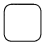# Math in Focus Grade 4 Chapter 3 Answer Key Whole Number Multiplication and Division

Go through the Math in Focus Grade 4 Workbook Answer Key Chapter 3 Whole Number Multiplication and Division to finish your assignments.

## Math in Focus Grade 4 Chapter 3 Answer Key Whole Number Multiplication and Division

Math Journal

Look at each problem. Use estimation to explain why the answers are not reasonable.

Example
5,268 × 8 = 2,144
Explain.
5,000 × 8 = 40,000
So the answer is too small.

Question 1.
725 × 6 = 700
Explain.
700 × 6 = 4,200
So the answer is too small.

Question 2.
497 × 21 = 1,291
Explain.
500×21 = 10,500.
So the answer is too small.

Use estimation to explain why the answer is not reasonable.

Question 3.
6,021 ÷ 3 = 207
Explain.
____
6,000 ÷ 3 = 2,000.
So the answer is too small.

Question 4.
Look at the number sentence.
72 ÷ 6 = 12
How would you use this to find the missing quotient?
7200 ÷ 6 =7200 ÷ 6 = 1200.

Explanation:
As 72 ÷ 6 = 12, so for 7200 ÷ 6 it will be 1200.

Challenging Practice

Charlie has 1,243 stamps. He gives away 12 stamps. His father gives him 415 stamps. He divides as many stamps as possible equally among 4 albums.

Question 1.
How many stamps did he place in each album?He places 411 stamps in each album.

Explanation:
Given that Charlie has 1,243 stamps and he gives away 12 stamps then it will be 1,243 – 12 = 1,231 and his father gives him 415 stamps then it will be 1,231+415 which is 1,646. As he divides as many stamps as possible equally among 4 albums it will be 1,646÷4 which will be approx 411 stamps.

Question 2.
Based on your answer in Exercise 1, how many stamps are left over?
The cost of 2 televisions and 3 DVD players is $1,421. The cost of 1 DVD player is half the cost of 1 television. What is the cost of 1 television? Answer: Explanation: Given that the cost of 2 televisions and 3 DVD players is$1,421 and the cost of 1 DVD player is half the cost of 1 television. Let the cost of 1 DVD player be X, so the price of the television will be 2X. So 2 televisions and 3 DVD player will be 2(2X)+3(X) = $1,421 4X+3X =$1,421
7X = $1,421 X =$203.
So the cost of 1 television it will be 2($203) which is$406.